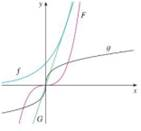Chapter 1.2, Problem 4E### Single Variable Calculus: Early Tr...

8th Edition
James Stewart
ISBN: 9781305270343

#### Solutions

Chapter
Section### Single Variable Calculus: Early Tr...

8th Edition
James Stewart
ISBN: 9781305270343
Textbook Problem

# Match each equation with its graph. Explain your choices. (Don't use a computer or graphing calculator.)4. (a) y = 3 x (b) y = 3 x (c) y = x 3 (d) y = x 3(a)

To determine

To match: The equation y=3x with its graphs without using a computer or graphing calculator.

Explanation

The equation y=3x is in the form of y=mx that represents the equation of straight line.

Here, the slope (m) of the straight line is 3 and the y-intercept is 0

(b)

To determine

To match: The equation y=3x with its graphs without using a computer or graphing calculator.

(c)

To determine

To match: The equation y=x3 with its graphs without using a computer or graphing calculator.

(d)

To determine

To match: The equation y=x3 with its graphs without using a computer or graphing calculator.

### Still sussing out bartleby?

Check out a sample textbook solution.

See a sample solution

#### The Solution to Your Study Problems

Bartleby provides explanations to thousands of textbook problems written by our experts, many with advanced degrees!

Get Started

#### In Exercises 107-120, factor each expression completely. 114. 3x2 4x 4

Applied Calculus for the Managerial, Life, and Social Sciences: A Brief Approach

#### In Problems 19-44, factor completely. 36.

Mathematical Applications for the Management, Life, and Social Sciences

#### Give another name for ABC not shown.

Elementary Geometry For College Students, 7e